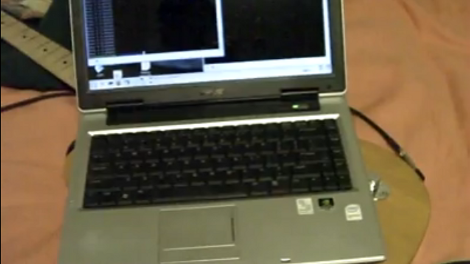# Using An Arduino To Measure InductanceMeasuring an inductor is not something that most multi-meters can do. You usually need a high precision resistor (1% or better) in series with the inductor, a function generator to put a signal through the circuit, and an oscilloscope to measure the result. But what can you do if you don’t have these tools on hand? [Andrew Moser] has a method that lets you pull it off with an Arduino and an LM339 quad comparator.

The circuit works by feeding a signal in from the Arduino. This waveform is affected by the LC circuit, filtered by the comparator chip, then read back out the other side by the Arduino. That resulting signal is a square wave, which is an easy target for the Arduino to measure. That timing measured from the square wave can then be used to calculate the inductor’s value.

This is quite handy if you’re winding your own inductors. Now you can precisely tune that Joule Thief you’ve been working on.